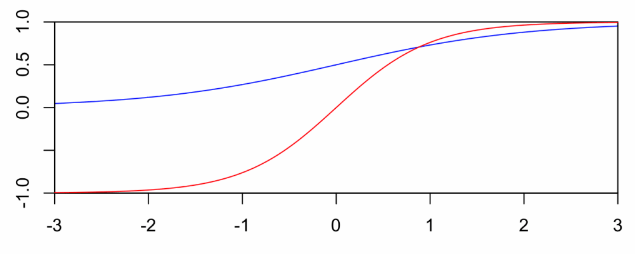#### LR中若标签为+1和-1，损失函数如何推导，求大佬解答

统计/机器学习 回归分析 监督式学习 损失函数    浏览次数：2742        分享

0GEASS   2018-09-05 10:08

##### 3个回答
4

logistic regression中用的是logistic sigmoid函数

$$\text{sigmoid}(x)=\frac{e^x}{e^x+1}\in [0,1]$$---------------------------------------------------------------------------------

$P(y_i|x_i)_w=\sigma(y_iz_i)$，$y_i=-1/1$, $z_i=w^Tx_i$

minimum negtive log likelihood可得损失函数：

$argmin_w{l(w)_{y,x}}$

$=argmin_w{-\sum_{i=1}^N ln\sigma(y_iz_i)}$

$=argmin_w{\sum_{i=1}^N ln\dfrac{1}{\sigma(y_iz_i)}}$

$=argmin_w{\sum_{i=1}^N ln(1+e^{-y_iz_i})}$

$argmin_w{CE(w)_{y,x}}$

$=argmin_w{-[t_i ln\sigma(z_i)+(1-t_i)ln(1-\sigma(z_i))]}$

$=argmin_w{-[(0.5y+0.5)ln\dfrac{1}{1+e^{-z_i}}+(0.5-0.5y)ln\dfrac{1}{1+e^{z_i}}]}$

$=argmin_w{ln(1+e^{z_i})-\dfrac{y+1}{2}z_i}$

$CE(w)_{y,x}=l(w)_{y,x}$

SofaSofa数据科学社区DS面试题库 DS面经Zealing   2018-09-05 13:15

2

SofaSofa数据科学社区DS面试题库 DS面经Jiho   2018-09-05 10:15

1

SofaSofa数据科学社区DS面试题库 DS面经ssssqd   2018-09-05 16:05相关主题